# Homology of complex projective space

This article describes the value (and the process used to compute it) of some homotopy invariant(s) for a topological space or family of topological spaces. The invariant is homology group and the topological space/family is complex projective space
Get more specific information about complex projective space | Get more computations of homology group

## Statement

### Unreduced version with coefficients in integers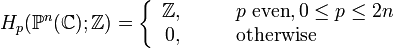$H_p(\mathbb{P}^n(\mathbb{C});\mathbb{Z}) = \left\lbrace\begin{array}{rl} \Z, & \qquad p \ \operatorname{even}, 0 \le p \le 2n\\ 0, & \qquad \operatorname{otherwise}\\\end{array}\right.$

### Reduced version with coefficients in integers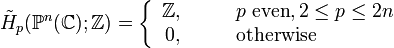$\tilde{H}_p(\mathbb{P}^n(\mathbb{C});\mathbb{Z}) = \left\lbrace\begin{array}{rl} \Z, & \qquad p \ \operatorname{even}, 2 \le p \le 2n\\ 0, & \qquad \operatorname{otherwise}\\\end{array}\right.$

### Unreduced version with coefficients in an abelian group or module

For coefficients in an abelian group$M$, the homology groups are:$H_p(\mathbb{P}^n(\mathbb{C});M) = \left\lbrace\begin{array}{rl} M, & \qquad p \ \operatorname{even}, 0 \le p \le 2n\\ 0, & \qquad \operatorname{otherwise}\\\end{array}\right.$

## Homology groups with integer coefficients in tabular form

We illustrate how the homology groups work for small values of$n$ (whereby the dimension of the corresponding complex projective space is$2n$). Note that for$p > 2n$, all homology groups are zero, so we omit those cells for visual clarity.$n$ Complex projective space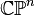$\mathbb{C}\mathbb{P}^n$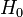$H_0$$H_1$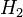$H_2$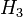$H_3$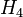$H_4$$H_5$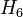$H_6$$H_7$$H_8$
1 2-sphere$\mathbb{Z}$ 0$\mathbb{Z}$
2 complex projective plane$\mathbb{Z}$ 0$\mathbb{Z}$ 0$\mathbb{Z}$
3 CP^3$\mathbb{Z}$ 0$\mathbb{Z}$ 0$\mathbb{Z}$ 0$\mathbb{Z}$
4 CP^4$\mathbb{Z}$ 0$\mathbb{Z}$ 0$\mathbb{Z}$ 0$\mathbb{Z}$ 0$\mathbb{Z}$

## Related invariants

These are all invariants that can be computed in terms of the homology groups.

Invariant General description Description of value for complex projective space$\mathbb{P}^n(\mathbb{C})$
Betti numbers The$k^{th}$ Betti number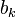$b_k$ is the rank of the torsion-free part of the$k^{th}$ homology group.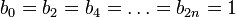$b_0 = b_2 = b_4 = \dots = b_{2n} = 1$, all other$b_k$ values are zero.
Poincare polynomial Generating polynomial for Betti numbers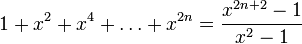$1 + x^2 + x^4 + \dots + x^{2n} = \frac{x^{2n + 2} - 1}{x^2 - 1}$
Euler characteristic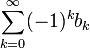$\sum_{k=0}^\infty (-1)^k b_k$$n + 1$

## Facts used

1. CW structure of complex projective space

## Proof

We use the CW-complex structure on complex projective space that has exactly one cell in every even dimension till$2n$. The cellular chain complex of this thus has$\Z$s in all the even positions till$2n$, and hence its homology is$\Z$ in all even dimensions till$2n$.